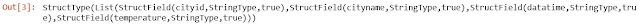# Linear Interpolation with Machine Learning | PySpark

## Linear Interpolation using PySpark

In this blog, we will discuss about the problem statement and its solution built using Spark with python (PySpark) and Python pandas UDF in Machine Learning (Linear Interpolation).

### Problem Statement:

Consider we have a weather data of a city for particular day. The data that is captured is observed to have a gap of one hour between each record ie, there is an entry in file on hourly basis. So, for any particular day we will have 24 entries in that input file. But if we need to do some analysis on this weather data, hourly data of just 24 entries may not be helpful. We need some mechanism to convert the 24 hourly entries into 1440 entries, one entry per minute which makes our analysis on weather data meaningful.

Sample entries in weather data for our illustration, Here we can notice raise in temperature between 9 am to 12 pm, where we have entries one hour once

The output that we expect is to fill in with entries for each minute, so for an hour we will see 60 records between 9 am to 10 am as shown in below diagram

We build a solution using existing function in pandas, along with PySpark. I recommend reader to try each code snippet in your spark configured machine to have a better understanding. If you need a guidance on installing spark in your windows machine, make use of "Guide to Install Spark"

### What is Linear Interpolation?

Linear Interpolation is a method which is used to insert a data or record in between within the give range. Linear interpolation is used in Weather data analytics, stock market pricing, etc., Say we have point A and B as shown in diagram below. Using interpolation we can plot an another point C in between A and B,

Mathematically, linear interpolation is framed as a formula given below, just for your information

### Solution to the statement:

Let us have quick hands-on session, to interpolate the data and fill in values. Before that let me give some glimpse on how we are achieving this.

We use python pandas UDF (vectorized udfs) "Grouped Map". Grouped Map is a pandas UDFs used with groupBy(), apply() that implements "split-apply-combine" rule to get the output. We should also have some knowledge in Apache Arrow. As a basic info, Apache Arrow is an in-memory columanar data format that is used to efficiently transfer data between JVM and python processes. For full information on pandas udf with pyspark, you can have a look at "Apache Arrow" . Let's get started, Open a fresh Jupyter notebook from anaconda and initiate SparkSession to use.

# Create SparkSession and import all required packages
from pyspark.sql import SparkSession,types
from pyspark.sql.functions import unix_timestamp, pandas_udf, PandasUDFType
from pyspark.sql.types import TimestampType, StructType
from operator import attrgetter

spark = SparkSession.builder.master("local")\
.appName('Interpolation')\
.getOrCreate()

### Input File:

The sample input file is prepared as shown above in the begining of this chapter and read this csv file through spark as spark Dataframe.

df_in.schema

We could notice the schema of file with all the column of the dataframe are of stringtypeInput Schema

But for, doing re-sampling and interpolation of time series data, we need to have atleast one column with numerical value and the datetime column should be of timestamp format. So we cast the temperature column into "double" and "datatime" to "timestamp".

df_out=df_in.withColumn("datatime",unix_timestamp("datatime", 'MM/dd/yyyy HH:mm').cast(TimestampType()))
df_out1=df_out.withColumn("temperature", df_in["temperature"].cast("double"))
df_out1.show(5,truncate=0)

Also, you can observe that the datatype of both temperature and datatime is changed as per our need.

### Coding:

Now comes the main part of program, we will create a function to get "Grouped map", and then apply resampling and interpolation on the dataframe.

def resample(schema, freq, timestamp_col = "datatime",**kwargs):
@pandas_udf(
StructType(sorted(schema, key=attrgetter("name"))),
PandasUDFType.GROUPED_MAP)
def _(pdf):
pdf.set_index(timestamp_col, inplace=True)
pdf=pdf.resample(freq).interpolate()
pdf.ffill(inplace=True)
pdf.reset_index(drop=False, inplace=True)
pdf.sort_index(axis=1, inplace=True)
return pdf
return _

Here, "pdf" is nothing but pandas data frame.

Note: This logic works under assumption that both input as well as interpolated output for a single cityid or grouped data can fit in memory of a single in a cluster.

Next step after declaring the function is to do split-apply-combine the Spark dataframe. Follow the below snippet to do the same. Here "60S" indicates the interpolation of data for every 60 seconds.

df_final=df_out1.groupBy("cityid","cityname").apply(resample(df_out1.schema, "60S"))

### Interpolated Output:

We got the interpolated output stored as spark dataframe, we can save this dataframe as a csv file or else to display the output interactively, we can use "df_final.show()". From the below output, we can see that that temperature for each minute is generated and ready for our analysis.

### Full program for your reference:

Hope you enjoyed learning a trick to do interpolation to the given dataset in Machine learning using PySpark.

Happy Learning!!!